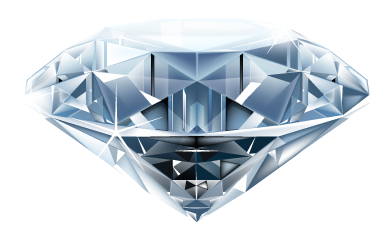# Illustrator Tutorial: Army CapIn this tutorial, you will learn how to design an army cap using Illustrator. In each step, we will first draw the outline and then apply colors and shadows. Finally, we will add the necessary details to complete the army cap. Let’s get started!

### Cap Body Design

1. Draw a simple sketch by using the Pen tool from the tool box.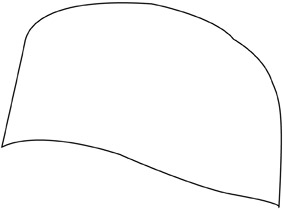2. Select the appropriate color (C=0, M=4, Y=25,K=0). This color makes good a base for the cap.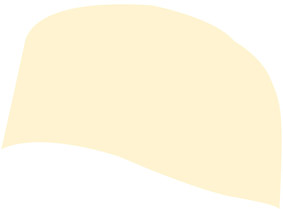3. Draw the leading edge of the cap with the Pen tool.4. Select the body color (C=0, M=4,Y=25, K=0).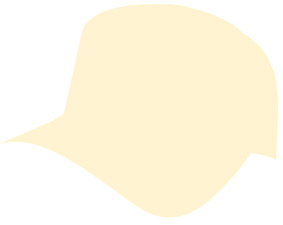5. Draw the cap’s leading edge seperately using the Pen tool.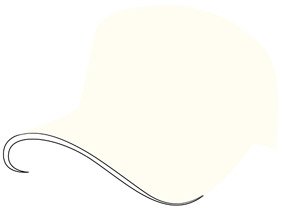6. Select the appropriate color (C=30, M=50, Y=75, K=10) and draw a midline to bold the cap’s leading ledge. Select the Stroke Adjustment Mode (as shown below).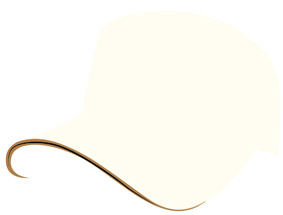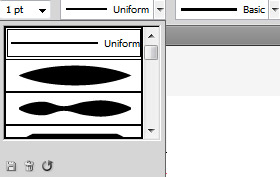7. Now, select the appropriate color (C=0, M=4, Y= 25, K=0) and then apply the Gussian blur from the Effect > Blur menu to add a blur effect.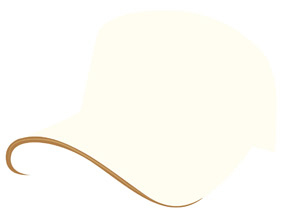8. Draw the bottom of the cap with the Pen tool.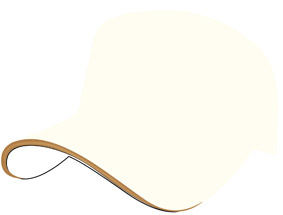9. Select the appropriate color (C=0, M 4, Y=25, K=0).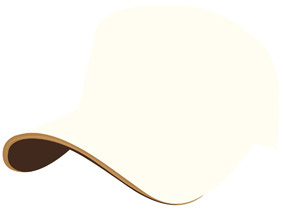10. Remove the stroke line.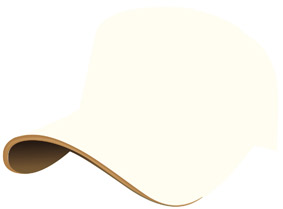11. Now, draw beyond the cap’s edge and button with the Pen tool.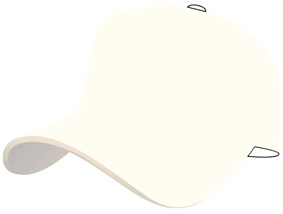12.Select (C=0, M=4, Y=25, K=0).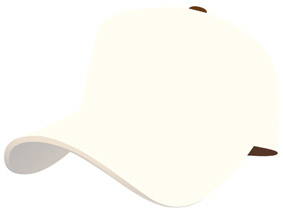13. You can use the gradient to add volume to the edges.14. Draw a line and change it (as we did in Step 6).15. Apply the Gussian blur from the Effect > Blur menu to add a blur effect.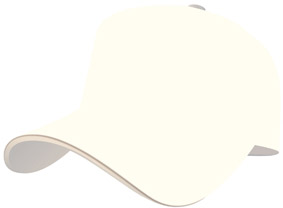16. Draw the camouflage textures that are on the cap using the pencil from the tool box. Draw these textures randomly. The final textures should create a nice camouflage pattern.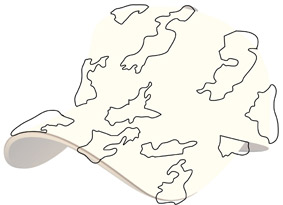17. Fill it with the appropriate color (C=39, M=25, Y=61, K=0).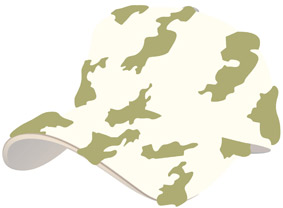18.Trim the textures protruding from the cap surroundings. In order to do this, you should first group all textures. Then, select the grouped textures with the cap object by holding the shift key. Apply the clipping mask from the Object > Clip Mask menu.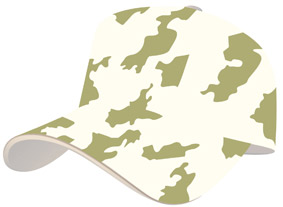19. For your cap to look better, you need to apply a linear gradient.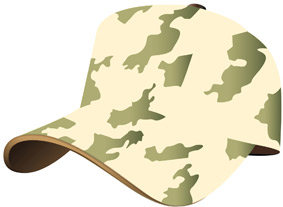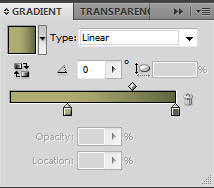20. For adding another color to the texture, draw some other objects with the Pen tool.21.Add an appropriate color (C=17, M=22, Y=36, K=0).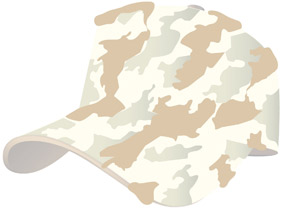22. Trim the protruding parts (as we did in Step 18).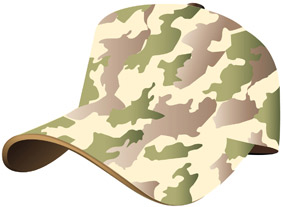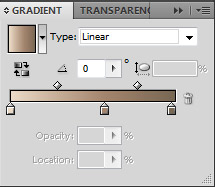24. For adding another color to the texture, draw more camouflage patterns with the Pen tool (as we did in Step 20).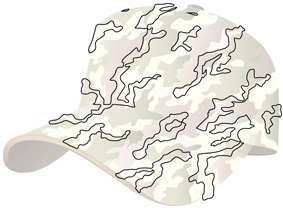25. Select the appropriate color (C=42, M=45, Y=56, K=9).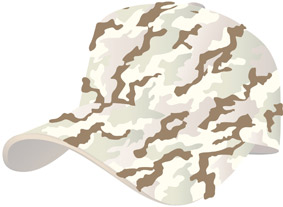26. Trim the protruding parts (as we did in Step 18).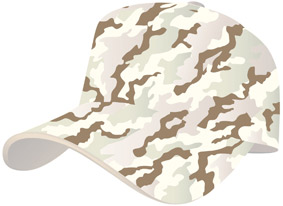27. Select the appropriate gradient color. To do this, the cap should appear like this.28. Draw the cap seams with the Pen tool.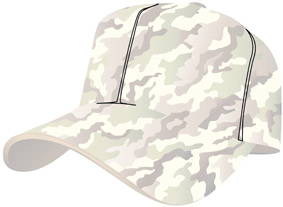29. Select the appropriate color (C=11, M=31, Y=38, K=0)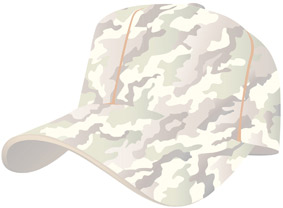30. Select “multiply blending” from the transparency palette. This step makes the seams look darker and more natural.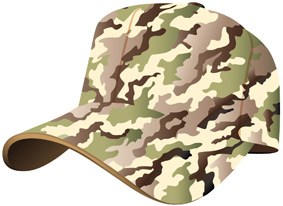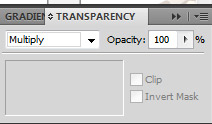31. Draw the cap sewing with the Pen tool.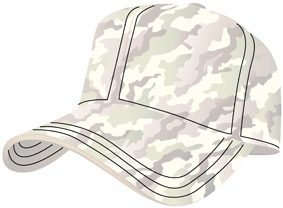32. Adjust the stroke setting as shown.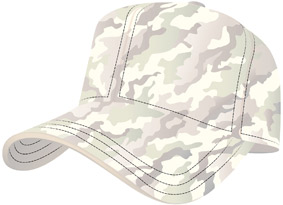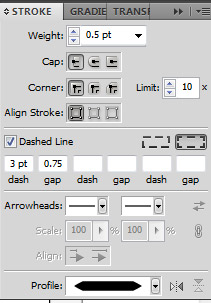33. Select the appropriate color (C=35, M=60, Y=80, K=25).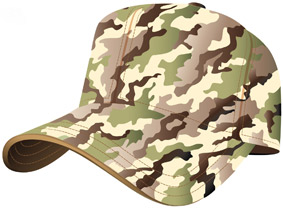34. Draw an object to create shadow above the cap’s cover.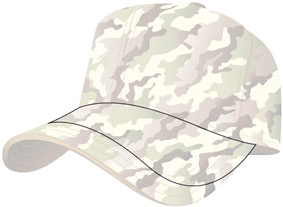35. Create a gradient as shown (left side colour-opacity=0). After we complete this action a part of the cap will be in shadow.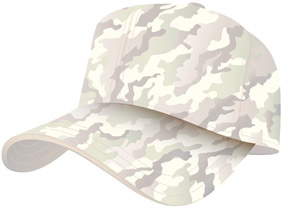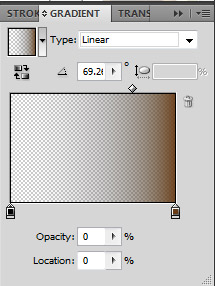36. By now, the cap shape should look like the one shown below.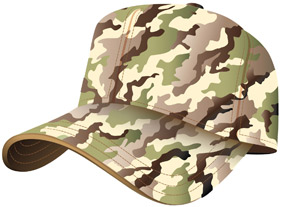37. Draw an object to make shadow on the right side.38. Fill drawn object with black color. You can also use gradient color (as shown).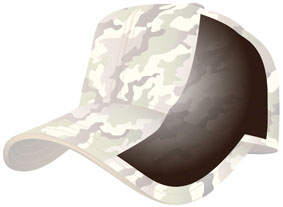39. Apply blur with Gussian blur from the Effect > Blur menu.40. Set Opacity to 57% from the transparency palette.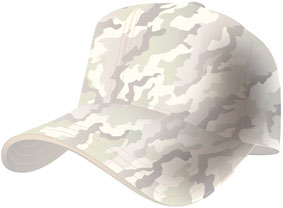41. Set multiply blending from the transparency palette to make the cap look more natural.42.Draw a line to create a sharp shadow.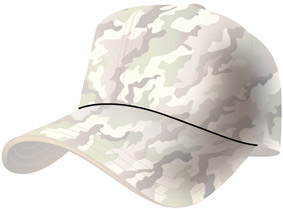43. Apply changes (as we did in Step 6).44.Select the appropriate color (C=40, M=70, Y=100, K=50).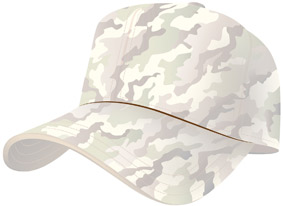45. Blur the object with Gussian blur from the Effect > Blur menu.46. Set the blur radius to 4 pixels in order to produce a better blur effect.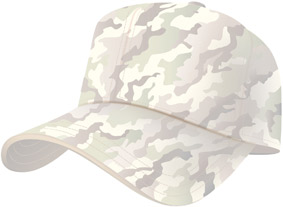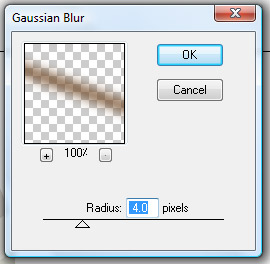47. Now the cap shape is complete. We will proceed to make the shadow below the cap.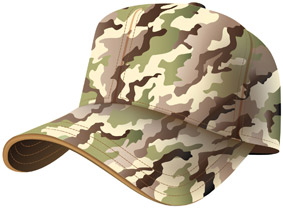48. To create a shadow, draw an object under the cap.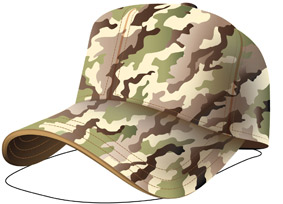49. Fill the drawn sketch with black color.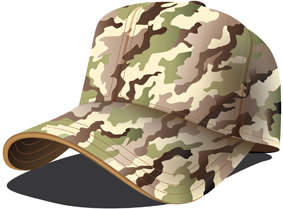50. Blur the shadow as many as 45-46 times and your camouflage pattern is completed.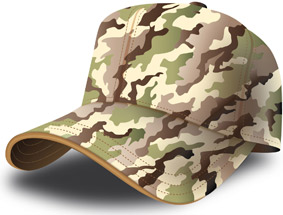[private_basic]
[/private_basic]

### Related Articles

See the list of all Illustrator tutorials.#### Related Articles

1.Ana Zivick May 1st

In general a good tutorial with a few gaps/mistakes:
step 7 didn’t work (I had to improvise another method)
step 9 and 12 and others – color code is incorrect (probably a copy/paste from step 4)
step 10 shows the bottom of cap with a gradient but doesn’t explain how that happened
there might be more..I have not yet finished the tutorial but a detailed revision by the author would help a lot.

2.3.